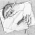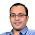### Wrong Solution

Source: Very interesting problem taken from Tanya Khovanova’s Math Blog

Problem:
I found this cute problem in the Russian book Sharygin Geometry Olympiad by Zaslavsky, Protasov and Sharygin.
Find numbers p and q that satisfy the equation: x2 + px + q = 0.
The book asks you to find a mistake in the following solution:
By Viète’s formulae we get a system of equations p + q = − p, pq = q. Solving the system we get two solutions: p = q = 0 and p = 1, q = −2.
What is wrong with this solution?

Update (26-05-2011)
Solution:
Posted by Sudeep Kamath (EECS PhD Student, UC Berkeley, EE IITB 2008 Alumnus) in comments and of course on Tanya Khovanova’s Math Blog

1.here, we are looking for the coefficients "p" & "q" most probably,
i think the question DOES NOT that
P & q ARE THE SOLUTIONS OF GIVEN QUADRATIC..

2.Very nice problem.

This approach misses out the case when p=q, so that you only need to enforce one root of the equation to be p. This gives the additional solution p=q=-1/2.

3.@Shaan. I did not understand your comment.

@Sudeep. \m/ \m/ I could not solve it, but was happy_max when I read the solution :) Thanks.

4.but when you put p=q=-1/2 in the equation the roots of the quadratic are coming out to be -1/2 and 1 which are distinct and we need the equal roots..

5.@satpal..
When you put p = q = -1/2,
The equation becomes 2x^2 - x - 1 = 0 and -1/2 is a root of this equation. The question never says that p and q are the only roots of the equation. :)

Its just a terminology difference. I would not have put this if this was not in Russian Olympiad book :)